# SUMIF multiple columns with one or several criteria in Excel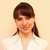by , updated on

This tutorial will teach you a few easy ways to sum multiple columns in Excel based on a single or multiple criteria.

Doing a conditional sum in Excel is a piece of cake as long as all the values to be totaled are in one column. Summing multiple columns is a problem because both the SUMIF and SUMIFS functions require the sum range and criteria ranges to be equally sized. Luckily, when there is no straight way to do something, there is always a work-around :)

## Excel Sum If: multiple columns, single criterion

First off, let's get to know exactly what problem we are trying to solve. Suppose you have a table of monthly sales like shown below. Because it was consolidated from a number of regional reports, there are a few records for the same product: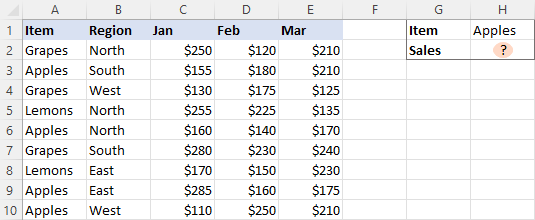The question is - how do you get a total of sales for a certain item?

The first idea that comes to mind is using a SUMIF formula in its pure form:

`=SUMIF(A2:A10, "apples", C2:E10)`

Unfortunately, this won't work. The reason is that the dimensions of sum_range are determined by Excel automatically based on the dimensions of the range argument. As our criteria range includes only one column (A2:A10), so does the sum range (C2:C10). The sum_range parameter defined in the formula (C2:E10) actually determines only the upper left cell of the range that will be summed. As a result, the above formula will add up the apples sales only in column C. Not what we are looking for, eh?

The simplest working solution that suggests itself is to create a helper column summing the numbers for each individual row, and then use that column for sum_range.

So, go ahead and place a SUM formula in F2, then drag it down across as many cells as needed:

`=SUM(C2:E2)`

After that, you can quickly have the job done:

`=SUMIF(A2:A10, I1, F2:F10)`

Where I1 is the item of interest.

In the above formula, sum_range is of the same size as range (1 column and 9 rows), so it works without a hitch: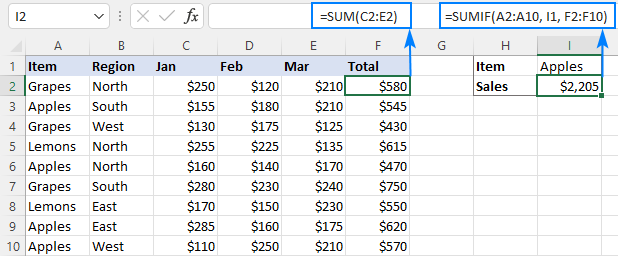If the layout of your worksheet does not have room for any extra columns, then apply one of the following solutions.

### SUMIF multiple columns

The idea is to write a separate SUMIF formula for each of the columns you want to sum, and then add up the results:

SUM(SUMIF(…), SUMIF(…), SUMIF(…))

Or

SUMIF(…) + SUMIF(…) + SUMIF(…)

A practical implementation looks as follows:

`=SUM(SUMIF(A2:A10,H1,C2:C10), SUMIF(A2:A10,H1,D2:D10), SUMIF(A2:A10,H1,E2:E10))`

Or

`=SUMIF(A2:A10, H1, C2:C10) + SUMIF(A2:A10, H1, D2:D10) + SUMIF(A2:A10, H1, E2:E10)`

You can also "hardcode" the condition in the formula if needed:

`=SUMIF(A2:A10, "Apples", C2:C10) + SUMIF(A2:A10, "Apples", D2:D10) + SUMIF(A2:A10, "Apples", E2:E10)`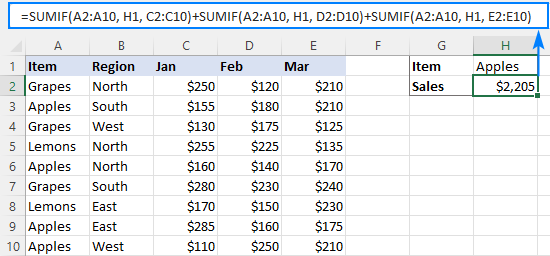This works fine for a reasonable number of columns, but for a large dataset the formula becomes too long and difficult to read. In this case, the below solutions are more appropriate.

### SUM as array formula

Another way to do a sum if in multiple columns based on one criterion is to construct an array formula:

SUM((sum_range) * (--(criteria_range=criteria)))

For our sample dataset, the formula takes this form:

`=SUM((C2:E10)*(--(A2:A10=H1)))`

Or

`=SUM((C2:E10)*(--(A2:A10="Apples")))`

In Excel 2019 and older, you should press Ctrl + Shift + Enter to complete the formula correctly. In Excel 365 and Excel 2021, this works as a normal formula due to inbuilt support for dynamic arrays.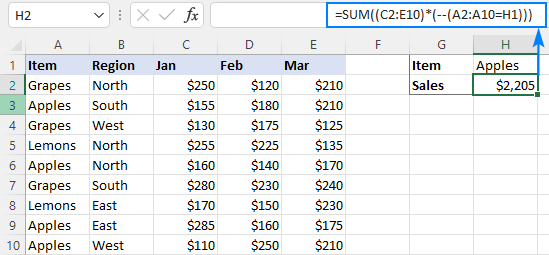How this formula works:

The core concept is to multiply the elements of these two arrays:

• (C2:E10) - all the values in the sum range. In our case, the array contains 27 elements (3 columns and 9 rows: {250,120,210;155,180,210;130,175,125; …}
• (--(A2:A10=H1)) - compares each value in A2:A10 against the target item in H1. The result is an array of TRUE (the condition is met) and FALSE (the condition is not met) values, which is then converted into an array of 1's and 0's with the help of a double unary operator: {0;1;0;0;1;0;0;1;1}

Please pay attention that the first array is two-dimensional (each column of data is separated by a comma and each row by a semicolon) and the second one is a one-dimensional vertical array (1 column of data, rows are separated by semicolons). When the two arrays are multiplied, all the items of the 2D array in a given row are multiplied by the corresponding element of the 1D array: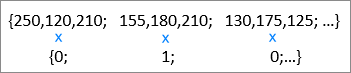As multiplying by zero gives zero, only the numbers for which the criterion is TRUE survive, and the SUM function adds them up:

`=SUM({0,0,0;155,180,210;0,0,0;0,0,0;160,140,170;0,0,0;0,0,0;…})`

To make the formula's logic easier to understand, you can write the first multiplier in this way:

`=SUM((C2:C10 + D2:D10 + E2:E10) * (--(A2:A10=H1)))`

This will produce an array of sums by row (like the helper column does in the very first example), which is then multiplied by an array of 1's and 0's:

`{580;545;430;615;470;750;550;620;570}*{0;1;0;0;1;0;0;1;1}`

The result of multiplication is served to SUM:

`=SUM({0;545;0;0;470;0;0;620;570})`

Don't like using arrays formulas in your sheet? Neither do I. Well, let's go check the next solution :)

### SUMPRODUCT formula

The strategy described in the above example can also be implemented using the SUMPRODUCT function.

SUMPRODUCT((sum_range) * (criteria_range=criteria))

A real-life formula goes as follows:

`=SUMPRODUCT((C2:E10) * (A2:A10=H1))`

The formula's logic is the same as in the previous example. The beauty of the SUMPRODUCT function is that it supports arrays natively, so it works nicely as a regular formula in all Excel versions.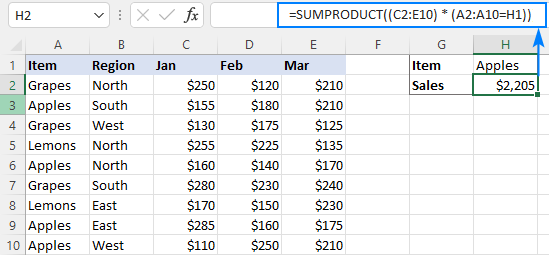## Excel Sum If: multiple columns, multiple criteria

The three approaches we utilized to add up multiple columns with one criterion will also work for conditional sum with multiple criteria. The formulas will just become a little more complex.

### SUMIFS + SUMIFS to sum multiple columns

To sum cells that match multiple criteria, you normally use the SUMIFS function. The problem is that, just like its single-criterion counterpart, SUMIFS doesn't support a multi-column sum range. To overcome this, we write a few SUMIFS, one per each column in the sum range:

SUM(SUMIFS(…), SUMIFS(…), SUMIFS(…))

Or

SUMIFS(…) + SUMIFS(…) + SUMIFS(…)

For example, to sum Grapes sales (H1) in the North region (H2), the formula is:

`=SUMIFS(C2:C10, A2:A10, H1, B2:B10, H2) + SUMIFS(D2:D10, A2:A10, H1, B2:B10, H2) + SUMIFS(E2:E10, A2:A10, H1, B2:B10, H2)`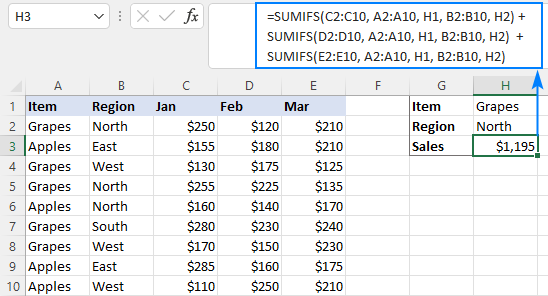### Array formula to conditionally sum multiple columns

The SUM formula for multiple criteria is very much like that for a single criterion - you just include additional criteria_range=criteria pair(s):

SUM((sum_range) * (--(criteria_range1=criteria1)) * (--(criteria_range2=criteria2)))

For instance, to sum sales for the item in H1 and the region in H2, the formula goes as follows:

`=SUM((C2:E10) * (--(A2:A10=H1)) * (--(B2:B10=H2)))`

In Excel 2019 and older, remember to press Ctrl + Shift + Enter to make it an CSE array formula. In dynamic array Excel 365 and 2021, a normal formula will work fine as shown in the screenshot: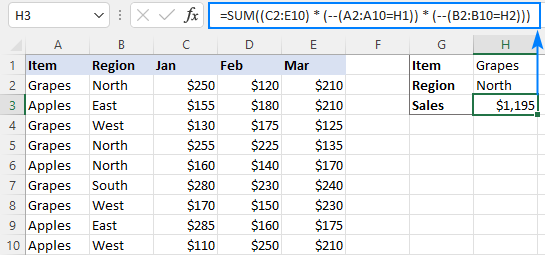### SUMPRODUCT formula with multiple criteria

The easiest way to sum multiple columns based on multiple criteria is the SUMPRODUCT formula:

SUMPRODUCT((sum_range) * (criteria_range1=criteria1) * (criteria_range2=criteria2))

As you can see, it's very similar to the SUM formula, but does not require any extra manipulations with arrays.

To sum multiple columns with two criteria, the formula is:

`=SUMPRODUCT((C2:E10) * (A2:A10=H1) * (B2:B10=H2))`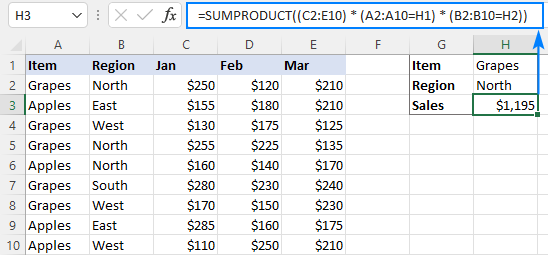These are the 3 ways to sum multiple columns based on one or more conditions in Excel. I thank you for reading and hope to see you on our blog next week!